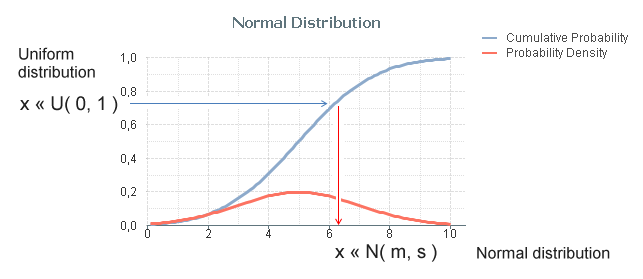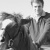# Qlik Design Blog

All about product and Qlik solutions: scripting, data modeling, visual design, extensions, best practices, etc.

Announcements
Read about the latest Qlik Community enhancements on the Community News blog!Employee

## Monte Carlo Methods

In some situations in Business Intelligence you need to make simulations, sometimes referred to as "Monte Carlo methods". These are algorithms that use repeated random number sampling to obtain approximate numerical results. In other words – using a random number as input many times, the methods calculate probabilities just like actually playing and logging your results in a real casino situation: hence the name.

These methods are used mainly to model phenomena with significant uncertainty in inputs, e.g. the calculation of risks, the prices of stock options, etc.

QlikView is very well suited for Monte Carlo simulations.

The basic idea is to generate data in the QlikView script using the random number generator Rand() in combination with a Load … Autogenerate, which generates a number of records without using an explicit input table.

To describe your simulation model properly, you need to do some programming in the QlikView script. Sometimes a lot. However, this is straightforward if you are used to writing formulae and programming code, e.g. Visual Basic scripts.

The Rand() function creates a uniformly distributed random number in the interval [0,1], which probably isn’t good enough for your needs: You most likely need to generate numbers that are distributed according to some specific probability density function. Luckily, it is in many cases not difficult to convert the result of Rand() to a random number with a different distribution.

The method used for this is called Inverse Transform Sampling: Basically, you take the cumulative probability function of the distribution, invert it, and use the Rand() function as input. See figure below.The most common probability distributions already exist in QlikView as inverse cumulative functions; Normal T, F and Chi-squared. Additional functions can be created with some math knowledge. The following definitions can be used for the most common distributions:

• Normal distribution: NormInv( Rand(), m, s )
• Log-Normal distribution: Exp( NormInv( Rand(), m, s ))
• Student's T-distribution: TInv( Rand(), d )
• F-distribution: FInv( Rand(), d1, d2 )
• Chi-squared distribution: ChiInv( Rand(), d )
• Exponential distribution: -m * Log( Rand() )
• Cauchy distribution: Tan( Pi() * (Rand()-0.5) )

Finally, an example that shows the principles around Monte Carlo methods: You want to estimate π (pi) using a Monte Carlo method. Then you could generate an arbitrary position x,y where both x and y are between 0 and 1, and calculate the distance to the origin. The script would e.g. be:

Sqrt(x*x + y*y) as r;

Rand() as x,

Rand() as y,

RecNo() as ID

Autogenerate 1000;The ratio between the number of instances that are within one unit of distance from the origin and the total number of instances should be π/4. Hence π can be estimated through 4*Count( If(r<=1, ID)) / Count(ID).

Bottom line: Should you need to make Monte Carlo simulations – don’t hesitate to use QlikView. You will be able to do quite a lot.

HICEmployee

I just got a question on Twitter concerning a Skewed Normal Distribution and want to answer here.

It is possible to create also this in the QlikView script, but you need to use preceding loads since you need to make the calculation in steps and reuse your result in several places.

Using vAlpha to denote the shape factor (see definition on Wikipedia) and vDelta, u0, u1 and ny to denote the corresponding parameters in the algorithm described here, you can generate such random numbers using:

Set vAlpha = 4 ;

Let vDelta = \$(#vAlpha)/sqrt(1+\$(#vAlpha)*\$(#vAlpha)) ;

if(u0>=0,1,-1)*u1 as [Skewed Normal];

\$(#vDelta)*u0+sqrt(1-sqr(\$(#vDelta)))*ny as u1;

NormInv(Rand(),0,1) as u0,

NormInv(Rand(),0,1) as ny

Autogenerate ... ;

HIC

3,536 ViewsNot applicable

Hi Henric,

I just posted question regarding something similar.

Can you plz put some light on my question here? Create and Save data in Qlikview

BR,

SAK

3,536 ViewsEmployee

Very interesting post, HIC.

Just one question.

Which dim/expression have you used to show those graphs?

3,536 ViewsEmployee

They are scatter charts with ID as dimension, i.e. one dot per value of ID. Further, they have two expressions: Avg(x) and Avg(y) that are used for the position of the dot. Finally, the colors of the dots are set by an expression: if(r<=1, RGB(20,20,20), RGB(180,180,180)).

See more on Recipe for Making a Scatter Chart.

HIC

3,536 ViewsEmployee

And Pi is infered by expression: 4*Count(if(r<=1,ID))/count(ID), right?

3,536 ViewsEmployee

Correct.

3,536 ViewsChampion III

Thanks Henric!

2,673 ViewsEmployee

Great blog post.  Also check out this free QlikView app on Monte Carlo posted here:  http://community.qlik.com/message/329612#329612

2,673 ViewsSpecialist

Thanks for this very interesting post.

Do you have business case or idea to use it ?

I have no idea how i can adopt it...

2,673 ViewsEmployee

One example is the QlikView ROI calculator. See more on this video QlikView ROI Calculator – 50 User Scenario.

HIC

2,673 Views